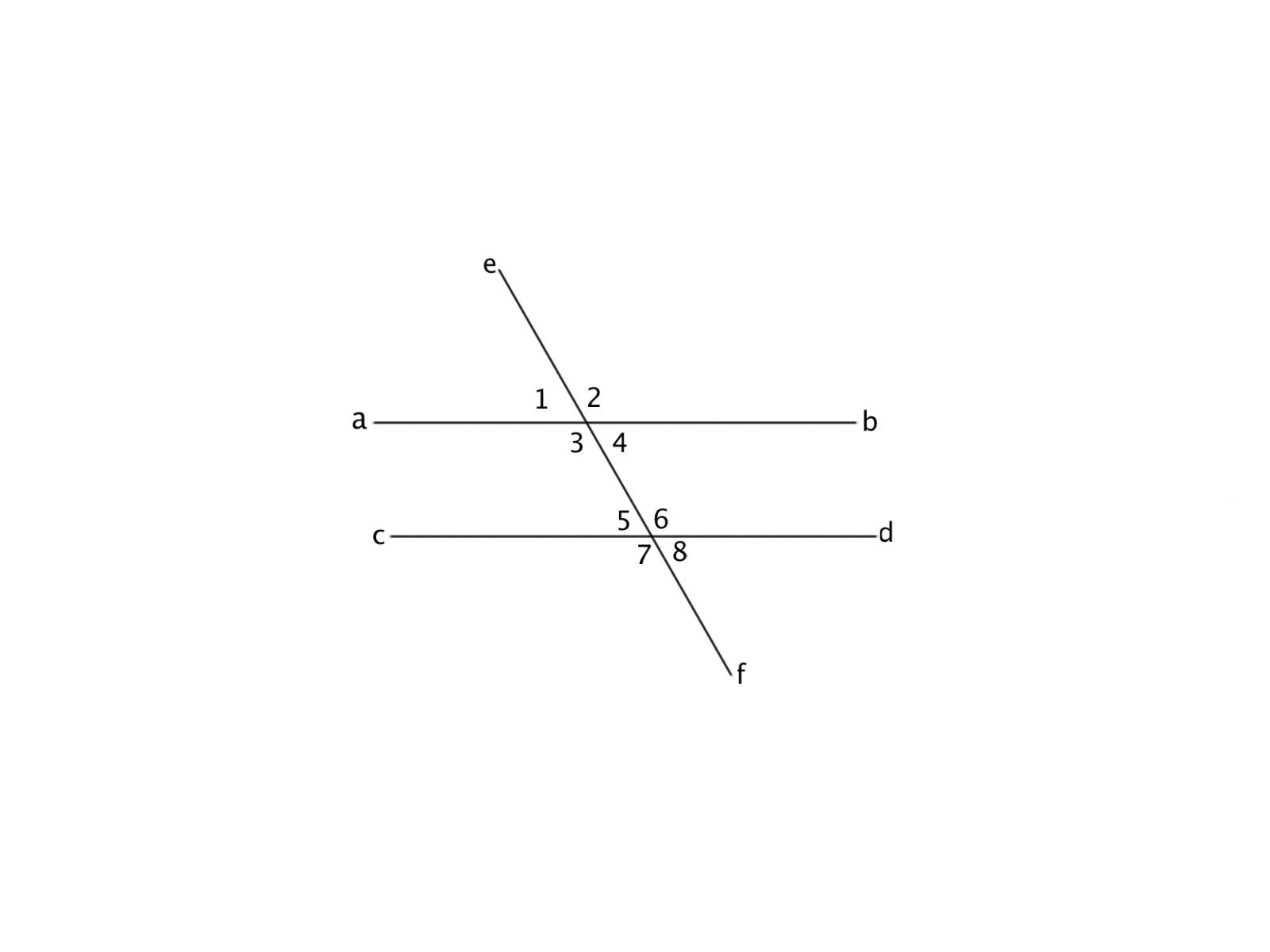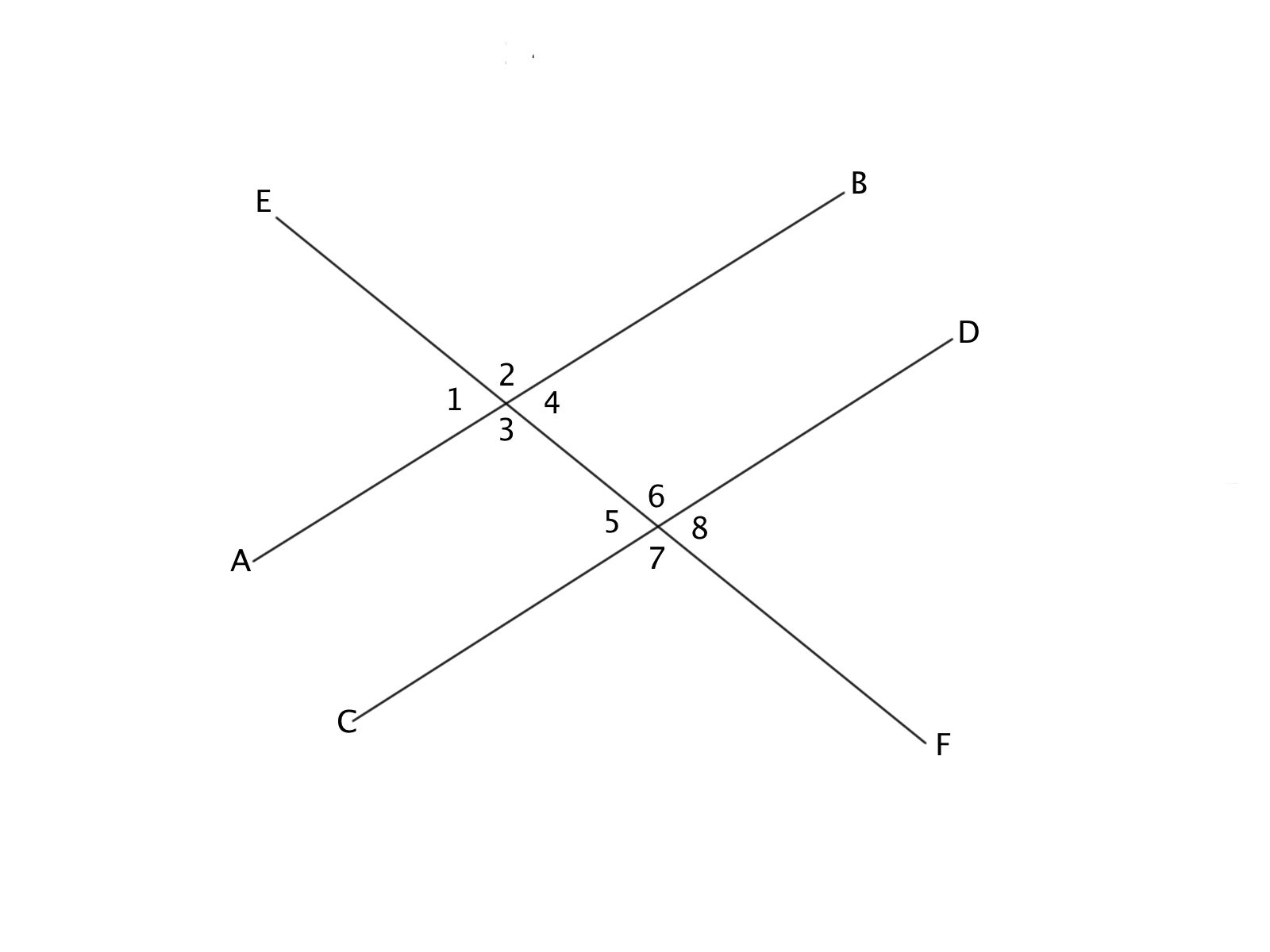# Intermediate Geometry : How to find out if lines are parallel

## Example Questions

← Previous 1

### Example Question #1 : How To Find Out If Lines Are ParallelWhich answer contains all the angles (other than itself) that are congruent to Angle 1?

Angles 8 and 6

Angles 2 and 4

Angles 4, 5, and 8

Angles 2 and 5

Angles 4 and 5

Angles 4, 5, and 8

Explanation:

Because of the Corresponding Angles Theorem (Angle 2 and Angle 5), Alternate Exterior Angles (Angle 2 and Angle 8), and Vertical Angles (Angle 2 and Angle 4).

### Example Question #1 : How To Find Out If Lines Are ParallelAngles 2 and 3 are congruent based on which Theorem?

Alternate Interior Angles

Consecutie Internior Angles

Corresponding Angles

Vertical Angles

Alternate Exteriors Angles

Vertical Angles

Explanation:

Veritcal angles means that the angles share the same vertex. Angles 2 and 3 are a vertical pair of angles, which mean that they are congruent.

### Example Question #1 : How To Find Out If Lines Are ParallelIf angles  2 and 6 are congruent, lines AB and CD are parallel based on which theorem?

Consecutive Interior Angles

Corresponding Angles

Alternate Interior Angles

Vertical Angles

Alternate Exterior Angles

Corresponding Angles

Explanation:

Angles 2 and 6 are Corresponding Angles. If each of the set of angles were taken separately, angels 2 and 6 would occupy the same place and are thus corresponding angles.

### Example Question #1 : How To Find Out If Lines Are ParallelWhat is the sum of Angle 3 and Angle 5?

90 deg

360 deg

15 deg

45 deg

180 deg

180 deg

Explanation:

Because of the Consecutive Interior Angle theorem, the sum of Angles 3 and 5 would be 180 deg.

### Example Question #1 : Parallel LinesIf lines AB and CD are parallel, angles 1 and 8 are congruent based on which theorem?

Alternate Exterior Angles

Consecutive Interior Angles

Corresponding Angles

Alternate Interior Angles

Vertical Angles

Alternate Exterior Angles

Explanation:

Angles 1 and 8 are on the exterior of the parallel lines and are on opposite sides of the transversal. This means the Theorem is the Alternate Exterior Angle theorem.

### Example Question #1 : How To Find Out If Lines Are ParallelIf Angles 2 and 7 are congruent, line AB and CD are __________.

skew

parallel

perpendicular

parallel

Explanation:

Lines AB and CD are parallel based on the Alternate Exterior Angle theorem.

### Example Question #1 : How To Find Out If Lines Are ParallelIf lines AB and CD are parallel, angles 5 and 1 are __________.

alternate exterior angles

corresponding angles

alternate interior angles

interior angles

exterior angles

corresponding angles

Explanation:

If the two lines are parallel, the transverse line makes it so that angles 2 and 7 are corresponding angles.

### Example Question #1 : Parallel LinesIf lines AB and CD are parallel, the sum of Angle 6 plus Ange 4 equals __________.

180 deg

0 deg

45 deg

90 deg

15 deg

180 deg

Explanation:

If lines AB and CD are parallel, the sum of Angles 4 and 6 is 180 deg based on the Consecutive Interior Angle Theorem.

### Example Question #1461 : Intermediate GeometryIf lines AB and CD are parallel, angles 2 and 7 are congruent based on which theorem?

There is not enough information to determine

Consecutive Angles

Corresponding Angles

Alternate Interior Angles

Alternate Exterior Angles

Alternate Exterior Angles

Explanation:

Angles 2 and 7 are both on the exterior side of the transverse, this means they are Alternate Exterior Angles.

### Example Question #1 : How To Find Out If Lines Are ParallelIf lines AB and CD are parallel, which angles are congruent to Angle 3?

Angles 5, 8, and 1

There is not enought information to determine

Angles 2, 7, and 6

Angles 7 and 6

Angles 1 and 5